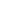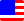Documents  >  Tutorial  >  group1
Operators of the first group

Purpose of this chapter.

We will learn about the method call operator, new operator, and this operator.

Operators of the first group.

Operators of the first group are following.

 () x(y) method call operator new new int() new operator this this this operator

method call operator

The method call operator is an operation in order to invoke a method.

As an example, let's call the ToString() method of the int class.

int i = 10;

string result = i.ToString() + " is 10."; // The result is "10 is 10."

The method call operator creates a signature from the method name and the argument. And, it calls an appropriate method.

As the above example, if there is a dot operator before the method name, the method call operator calls the method of the instance returned by the expression on the left side of the dot operator. Otherwise, the method call operator calls a method of the current instance.

A method call as an expression must return an instance. An exception will be thrown if you call a method with no return value as an expression.

new operator

The new operator is an operation in order to obtain an instance of the class specified by the fitter. The instance retrieved will be initialized with a fitter.

As an example, let's obtain an instance of the Circle class.

Circle cir = new Circle(10); // An instance with 10px radius.

You can get an instance of the desired class since the fitter name is the same as the class name.

In the above example, an instance of the Circle class with the radius set to 10px is returned. This instance has been properly initialized. However, it depends on the fitter whether the instance is initialized properly.

For example, the Circle class does not explicitly define a default fitter. Therefore, the default fitter is defined automatically by the system.

Circle cir = new Circle(); // This is default fitter. It returns An instance with 0px radius.

The default fitter defined by the system does not set the radius. Therefore, an instance of the Circle class with 0px radius is returned. This is not an instance initialized properly.

this operator

The this operator is an operation in order to get the current instance.